Courses

# Bessel's Special Function (Part - 1) - Mathematical Methods of Physics, UGC - NET Physics Physics Notes | EduRev

## Physics for IIT JAM, UGC - NET, CSIR NET

Created by: Akhilesh Thakur

## Physics : Bessel's Special Function (Part - 1) - Mathematical Methods of Physics, UGC - NET Physics Physics Notes | EduRev

The document Bessel's Special Function (Part - 1) - Mathematical Methods of Physics, UGC - NET Physics Physics Notes | EduRev is a part of the Physics Course Physics for IIT JAM, UGC - NET, CSIR NET.
All you need of Physics at this link: Physics

BESSEL FUNCTIONS OF THE FIRST KIND, Jν (x )

Bessel functions appear in a wide variety of physical problems. Separation of the Helmholtz, or wave, equation in circular cylindrical coordinates led to Bessel’s equation.  we will see that the Helmholtz equation in spherical polar coordinates also leads to a form of Bessel’s equation. Bessel functions may also appear in integral form — integral representations. This may result from integral transforms or from the mathematical elegance of starting the study of Bessel functions with Hankel functions.
Bessel functions and closely related functions form a rich area of mathematical analysis with many representations, many interesting and useful properties, and many interrelations.

Generating Function for Integral Order

Although Bessel functions are of interest primarily as solutions of differential equations, it is instructive and convenient to develop them from a completely different approach, that of the generating function.1 This approach also has the advantage of focusing on the functions themselves rather than on the differential equations they satisfy. Let us introduce a function of two variables,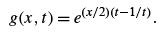(11.1)

Expanding this function in a Laurent series, we obtain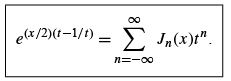(11.2)

It is instructive to compare Eq. (11.2) with the equivalent Eqs. (11.23) and (11.25).
The coefﬁcient of tn ,Jn (x ), is deﬁned to be a Bessel function of the ﬁrst kind, of integral order n. Expanding the exponentials, we have a product of Maclaurin series in xt /2 and −x/2t , respectively,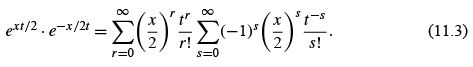Here, the summation index r is changed to n, with n = r − s and summation limits n =−s to ∞, and the order of the summations is interchanged, which is justiﬁed by absolute convergence. The range of the summation over n becomes −∞ to ∞, while the summation over s extends from max(−n, 0) to ∞. For a given s we get tn (n ≥ 0) from r = n + s :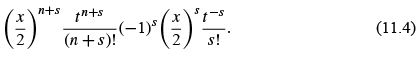The coefﬁcient of tn is then 2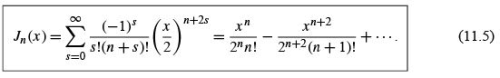This series form exhibits is behavior of the Bessel function Jn (x ) for small x and permits numerical evaluation of Jn (x ). The results for J0 ,J1 , and J2 are shown in Fig. 11.1. the error in using only a ﬁnite number of terms of this alternating series in numerical evaluation is less than the ﬁrst term omitted. For instance, if we want Jn (x )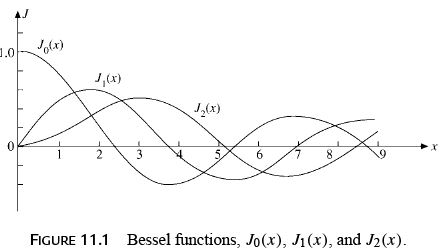to ±1% accuracy, the ﬁrst term alone of Eq. (11.5) will sufﬁce, provided the ratio of the second term to the ﬁrst is less than 1% (in magnitude) or x< 0.2(n + 1)1/2 . The Bessel functions oscillate but are not periodic — except in the limit as x →∞ (Section 11.6). The amplitude of Jn (x ) is not constant but decreases asymptotically as x −1/2 . (See Eq.(11.137) for this envelope.)

For n< 0, Eq. (11.5) gives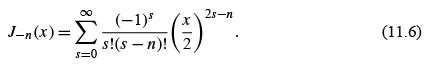Since n is an integer (here), (s − n)!→∞ for s = 0,...,(n − 1). Hence the series may be considered to start with s = n. Replacing s by s + n, we obtain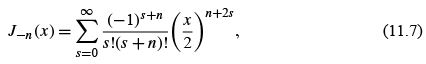showing immediately that Jn (x ) and J−n (x ) are not independent but are related by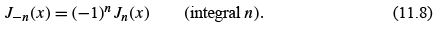These series expressions (Eqs. (11.5) and (11.6)) may be used with n replaced by ν to deﬁne Jν (x ) and J−ν (x ) for nonintegral ν (compare Exercise 11.1.7).

Recurrence Relations

The recurrence relations for Jn (x ) and its derivatives may all be obtained by operating on the series, Eq. (11.5), although this requires a bit of clairvoyance (or a lot of trial and error). Veriﬁcation of the known recurrence relations is straightforward, Exercise 11.1.7.
Here it is convenient to obtain them from the generating function, g(x , t ). Differentiating both sides of Eq. (11.1) with respect to t , we ﬁnd that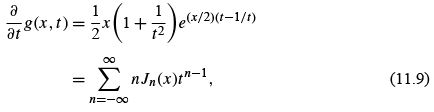and substituting Eq. (11.2) for the exponential and equating the coefﬁcients of like powers of t ,3 we obtain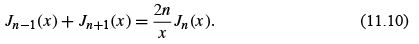This is a three-term recurrence relation. Given J0 and J1 , for example, J2 (and any other integral order Jn ) may be computed.

Differentiating Eq. (11.1) with respect to x ,wehave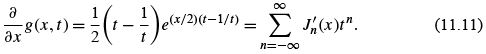Again, substituting in Eq. (11.2) and equating the coefﬁcients of like powers of t , we obtain the result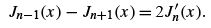(11.12)

As a special case of this general recurrence relation,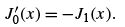(11.13)

Adding Eqs. (11.10) and (11.12) and dividing by 2, we have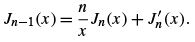(11.14)

Multiplying by x n and rearranging terms produces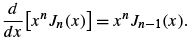(11.15)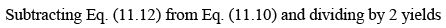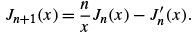(11.16)

Multiplying by x −n and rearranging terms, we obtain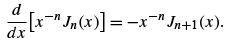(11.17)

Bessel’s Differential Equation

Suppose we consider a set of functions Zν (x ) that satisﬁes the basic recurrence relations (Eqs. (11.10) and (11.12)), but with ν not necessarily an integer and Zν not necessarily given by the series (Eq. (11.5)). Equation (11.14) may be rewritten (n → ν) as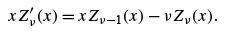(11.18)

On differentiating with respect to x ,wehave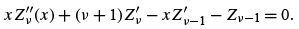(11.19)

Multiplying by x and then subtracting Eq. (11.18) multiplied by ν gives us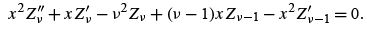(11.20)

Now we rewrite Eq. (11.16) and replace n by ν − 1: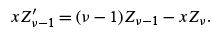(11.21)

Using Eq. (11.21) to eliminate Zν −1 and Zν −1 from Eq. (11.20), we ﬁnally get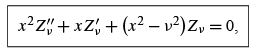(11.22)

which is Bessel’s ODE. Hence any functions Zν (x ) that satisfy the recurrence relations (Eqs. (11.10) and (11.12), (11.14) and (11.16), or (11.15) and (11.17)) satisfy Bessel’s equation; that is, the unknown Zν are Bessel functions. In particular, we have shown that the functions Jn (x ), deﬁned by our generating function, satisfy Bessel’s ODE. If the argument is kρ rather than x , Eq. (11.22) becomes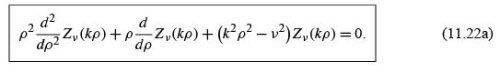Integral Representation

A particularly useful and powerful way of treating Bessel functions employs integral representations. If we return to the generating function (Eq. (11.2)), and substitute t = e ,we get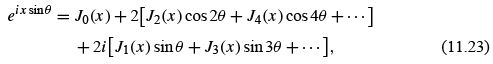in which we have used the relations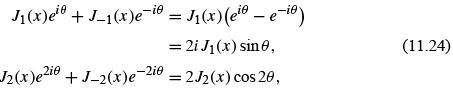and so on.
In summation notation,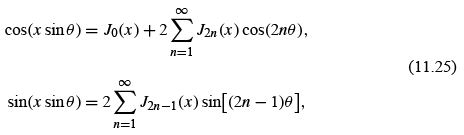equating real and imaginary parts of Eq. (11.23).
By employing the orthogonality properties of cosine and sine,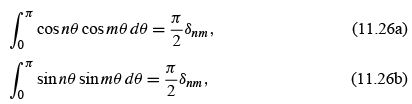in which n and m are positive integers (zero is excluded), we obtain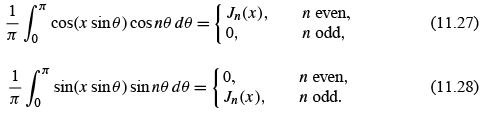If these two equations are added together,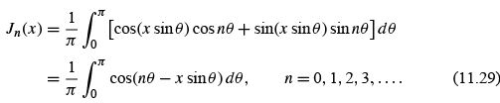As a special case (integrate Eq. (11.25) over (0,π ) to get)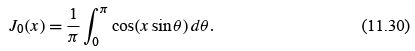Noting that cos(x sin θ) repeats itself in all four quadrants, we may write Eq. (11.30) as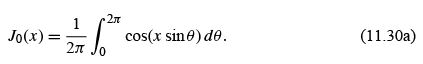On the other hand, sin(x sin θ) reverses its sign in the third and fourth quadrants, so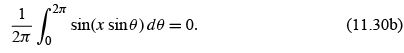Adding Eq. (11.30a) and i times Eq. (11.30b), we obtain the complex exponential representation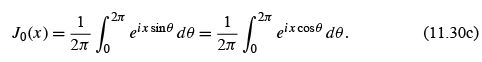This integral representation (Eq. (11.29)) may be obtained somewhat more directly by employing contour integration (compare Exercise 11.1.16).6 Many other integral representations exist (compare Exercise 11.1.18).

Example 11.1.1 FRAUNHOFER DIFFRACTION,CIRCULAR APERTURE

In the theory of diffraction through a circular aperture we encounter the integral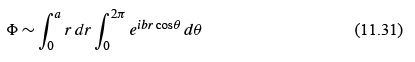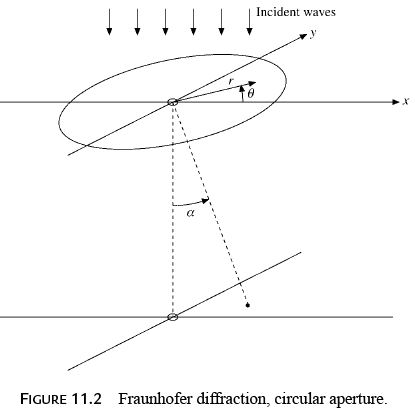for φ, the amplitude of the diffracted wave. Here θ is an azimuth angle in the plane of the circular aperture of radius a , and α is the angle deﬁned by a point on a screen below the circular aperture relative to the normal through the center point. The parameter b is given by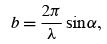(11.32)

with λ the wavelength of the incident wave. The other symbols are deﬁned by Fig. 11.2.
From Eq. (11.30c) we get8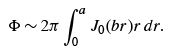(11.33)

Equation (11.15) enables us to integrate Eq. (11.33) immediately to obtain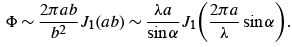(11.34)

Note here that J1 (0) = 0. The intensity of the light in the diffraction pattern is proportional to φand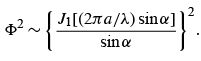(11.35)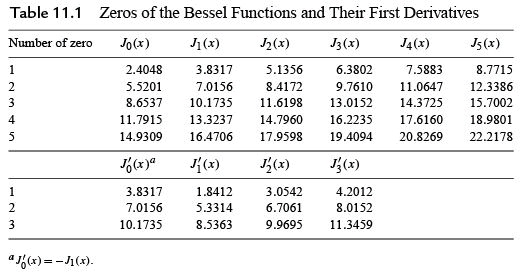From Table 11.1, which lists the zeros of the Bessel functions and their ﬁrst derivatives,  Eq. (11.35) will have a zero at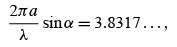(11.36)

or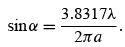(11.37)

For green light, λ = 5.5 × 10−5 cm. Hence, if a = 0.5cm,

α ≈ sin α = 6.7 × 10−5 (radian) ≈ 14 seconds of arc, (11.38)

which shows that the bending or spreading of the light ray is extremely small. If this analysis had been known in the seventeenth century, the arguments against the wave theory of light would have collapsed. In mid-twentieth century this same diffraction pattern appears in the scattering of nuclear particles by atomic nuclei — a striking demonstration of the wave properties of the nuclear particles.

A further example of the use of Bessel functions and their roots is provided by the electromagnetic resonant cavity (Example 11.1.2) and the example and exercises of Section 11.2.

Example 11.1.2 CYLINDRICAL RESONANT CAVITY

The propagation of electromagnetic waves in hollow metallic cylinders is important in many practical devices. If the cylinder has end surfaces, it is called a cavity. Resonant cavities play a crucial role in many particle accelerators.

11.1 Bessel Functions of the First Kind, Jν (x )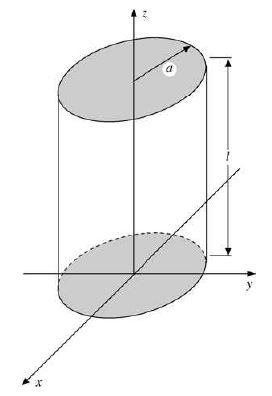FIGURE 11.3 Cylindrical resonant cavity.

We take the z-axis along the center of the cavity with end surfaces at z = 0 and z = l and use cylindrical coordinates suggested by the geometry. Its walls are perfect conductors, so the tangential electric ﬁeld vanishes on them (as in Fig. 11.3):

Ez = 0 = Eϕ for ρ = a, Eρ = 0 = Eϕ for z = 0,l.

Inside the cavity we have a vacuum, so ε0 µ0 = 1/c2 . In the interior of a resonant cavity, electromagnetic waves oscillate with harmonic time dependence e−iωt , which follows from separating the time from the spatial variables in Maxwell’s equations (Section 1.9), so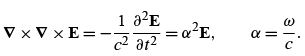With ∇ · E = 0 (vacuum, no charges) and Eq. (1.85), we obtain for the space part of the electric ﬁeld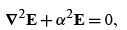which is called the vector Helmholtz PDE.The z-component ( Ez , space part only) satisﬁes the scalar Helmholtz equation,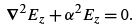(11.39)

The transverse electric ﬁeld components E⊥ = (Eρ ,Eϕ ) obey the same PDE but different boundary conditions, given earlier. Once Eis known, Maxwell’s equations determine Eϕ fully.This implies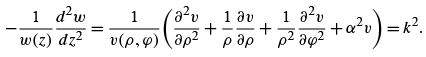Here, kis a separation constant, because the left- and right-hand sides depend on different variables. For w(z) we ﬁnd the harmonic oscillator ODE with standing wave solution (not transients) that we seek,

w(z) = A sin kz + B cos kz,

with A, B constants. For v(ρ , ϕ ) we obtain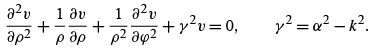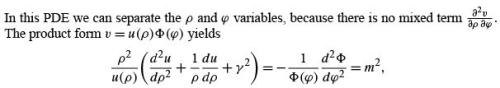where the separation constant m2 must be an integer, because the angular solution φ = eimϕ of the ODE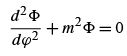must be periodic in the azimuthal angle.
This leaves us with the radial ODE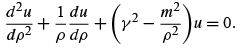Dimensional arguments suggest rescaling ρ → r = γρ and dividing by γ2 , which yields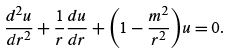This is Bessel’s ODE for ν = m. We use the regular solution Jm (γ ρ ) because the (irregular) second independent solution is singular at the origin, which is unacceptable here. The complete solution is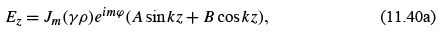where the constant γ is determined from the boundary condition Ez = 0 on the cavity surface ρ = a, that is, that γa be a root of the Bessel function Jm (see Table 11.1). This gives a discrete set of values γ = γmn , where n designates the nth root of J(see Table 11.1).

For the transverse magnetic or TM mode of oscillation with Hz = 0 Maxwell’s equations imply. (See again Resonant Cavities in J. D. Jackson’s Electrodynamics in Additional Readings.)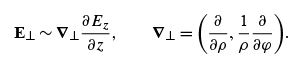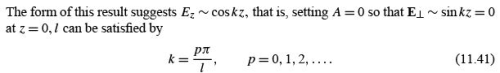with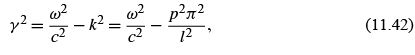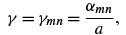(11.43)

where αmn is the nth zero of Jm . The general solution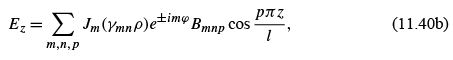with constants Bmnp , now follows from the superposition principle.
The result of the two boundary conditions and the separation constant m2 is that the angular frequency of our oscillation depends on three discrete parameters: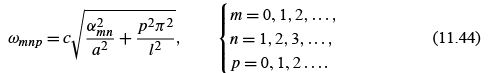These are the allowable resonant frequencies for our TM mode.

Alternate Approaches

Bessel functions are introduced here by means of a generating function, Eq. (11.2). Other approaches are possible. Listing the various possibilities, we have:

1. Generating function (magic), Eq. (11.2).
2. Series solution of Bessel’s differential equation,
3. Contour integrals: Some writers prefer to start with contour integral deﬁnitions of the Hankel functions, and develop the Bessel function Jν (x) from the Hankel functions.
4. Direct solution of physical problems: Example 11.1.1. Fraunhofer diffraction with a circular aperture, illustrates this. Incidentally, Eq. (11.31) can be treated by series expansion, if desired. Feynman10 develops Bessel functions from a consideration of cavity resonators.

In case the generating function seems too arbitrary, it can be derived from a contour integral,  or from the Bessel function recurrence relations,
Note that the contour integral is not limited to integer ν , thus providing a starting point for developing Bessel functions.

159 docs

,

,

,

,

,

,

,

,

,

,

,

,

,

,

,

,

,

,

,

,

,

,

,

,

;